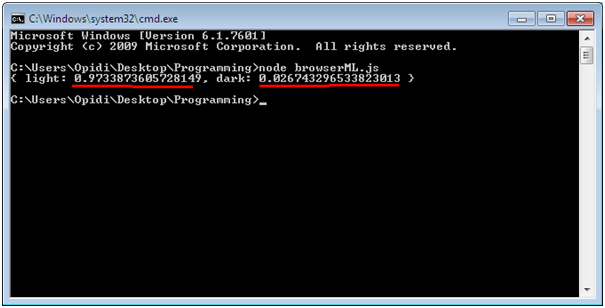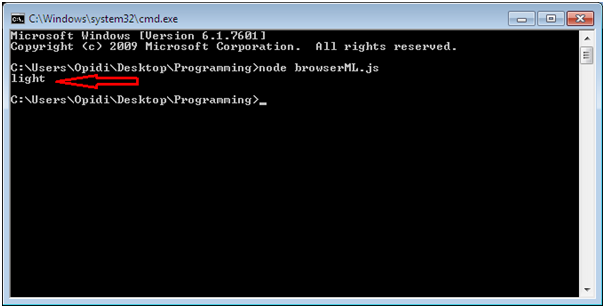{{announcement.body}}
{{announcement.title}}

# A Simple Machine Learning Project in JavaScript

DZone 's Guide to

# A Simple Machine Learning Project in JavaScript

### This tutorial teaches you how to use Javascript to define, train, and deploy machine learning algorithms completely in the browser.

· AI Zone ·
Free Resource

Comment (0)

Save
{{ articles.views | formatCount}} Views

Machine learning in the web browser?

Yes, it’s possible to use JavaScript to define, train, and deploy machine learning algorithms completely in the browser.

Although it may not be a conventional selection for machine learning, JavaScript is proving to be capable of completing such tasks — even if it cannot compete with the principal machine learning language: Python.

Before going further, let’s do a little introduction to machine learning.

According to Skyhoshi, who is an experienced developer with over 13 years of experience, machine learning endows computers with the ability to learn from the provided data, identify patterns, and then draw conclusions without requiring explicit human intervention.

Traditionally, JavaScript is not commonly used in machine learning for several reasons, including limited library support and implementation complexities.

Nonetheless, JavaScript has gained popularity recently mainly because of the extensive opportunities available in deploying machine learning applications entirely on the client-side.

In this article, I’m going to illustrate a simple machine learning tutorial project that demonstrates that JavaScript is also capable of powering machine learning applications.

## Let’s Get Started

For this simple project, I’m going to use brain.js, which is a handy open source JavaScript library with pre-made neural networks for machine learning.

The objective of this project is to train a neural network with a set of training data such that it can predict whether a color contrast is light or dark.

Step 1: Install the library

Let’s use Node.js to install the library using the npm package manager. For this to work, you need to have Node.js pre-installed on your computer.

Here is the code you can run on your terminal. (Remember to install it on the folder you’ll be working on).

`npm install brain.js`

Step 2: Import the library

Import the library on your file using the following code:

`const brain = require("brain.js");`

Step 3: Create a neural network

Here is the code:

`const network = new brain.NeuralNetwork();`

Step 4: Train the data

In machine learning, the training data is as good as the outcome that will be received. A higher quality data is likely to predict better outcomes than a lower quality data.

This data is a set of examples that is provided to an algorithm to “instruct” it about what to search for.

In this case, since we want our machine learning algorithm to learn to identify the difference between light and dark color contrasts, we will give it those examples to train the model.

Thereafter, the algorithm will employ the provided examples to discern the essential characteristics in differentiating between the two groups.

If provided with unknown data in the future, the algorithm will classify according to the features it recognizes from the originally trained model.

For this project, we are going to use the in-built brain.js train() function to train our neural network using an array of example data.

Every example training data will have an input object and an output object, of which both should be an array of numbers from 0 to 1.

Since we are working with colors, we are going to go for their RGB values. Since RGB colors are between 0 and 255, we can convert them to have their values between 0 and 1 by dividing by 255.

For example, if the RGB value of a color is (158,183,224). If we convert each of the values by dividing by 255, it will become (0.62, 0.72,0.88).

Thereafter, we need to provide some example dataset of RBG values and specify whether the output will be light or dark.

Here is the code:

``````network.train([

{input: {r:0.62,g:0.72,b:0.88}, output:{light: 1}},

{input: {r:0.1,g:0.84,b:0.72}, output:{light: 1}},

{input: {r:0.33,g:0.24,b:0.29}, output:{dark: 1}},

{input: {r:0.74,g:0.78,b:0.86}, output:{light: 1}},

]);``````

Step 5: View results

After training the algorithm with some example data, it’s now time to view the prediction results.

Are you excited?

We just call the run() function and provide an argument of the color hue we would like to know whether is light or dark.

Here is the code:

``````const result = network.run({r:0.0,g:1,b:0.65});

console.log(result);``````

If we execute it on the Windows terminal, the output will be something like this:As you can see, our machine learning for beginners algorithm predicted that the color contrast is light with an accuracy of 0.97 (97%).

If we want the output to be either light or dark, we can add the following code:

``````const result = brain.likely({r:0.0,g:1,b:0.65}, network);

console.log(result);``````

Here is the result on the terminal.## Conclusion

Here is the code for the entire project:

``````const brain = require("brain.js");

const network = new brain.NeuralNetwork();

network.train([

{input: {r:0.62,g:0.72,b:0.88}, output:{light: 1}},

{input: {r:0.1,g:0.84,b:0.72}, output:{light: 1}},

{input: {r:0.33,g:0.24,b:0.29}, output:{dark: 1}},

{input: {r:0.74,g:0.78,b:0.86}, output:{light: 1}},

]);

//const result = network.run({r:0.0,g:1,b:0.65});

const result = brain.likely({r:0.0,g:1,b:0.65}, network);

console.log(result);``````

In this post, I demonstrated a simple machine learning project in JavaScript. To improve your machine learning skills, you need to complete such projects.

Happy learning machine learning!

Topics:
ai, ai artificial intelligence, artificial intelligence, javascript, machine learning, machine learning algorithms, node.js, python

Comment (0)

Save
{{ articles.views | formatCount}} Views

Published at DZone with permission of Dr. Michael Garbade . See the original article here.

Opinions expressed by DZone contributors are their own.